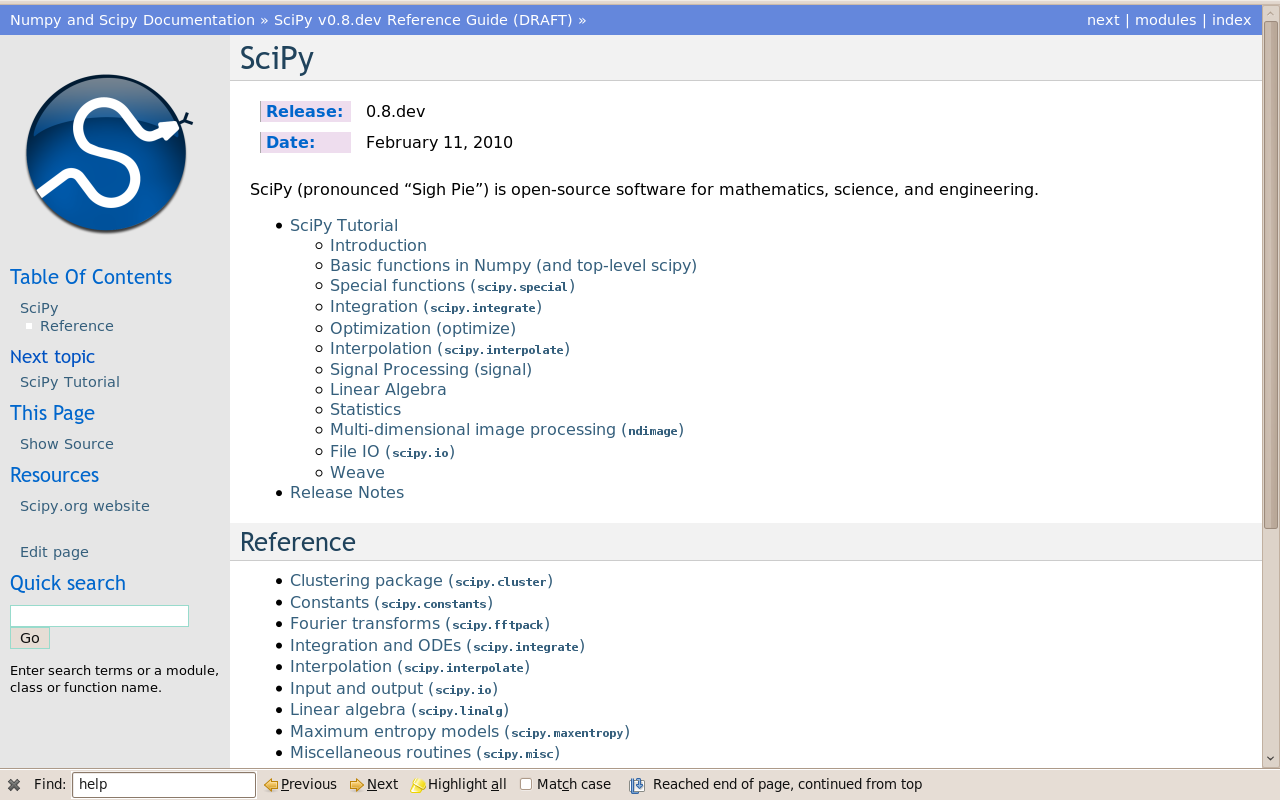# 1.6. Getting help and finding documentation¶

Author: Emmanuelle Gouillart

Rather than knowing all functions in Numpy and Scipy, it is important to find rapidly information throughout the documentation and the available help. Here are some ways to get information:

• In Ipython, help function opens the docstring of the function. Only type the beginning of the function’s name and use tab completion to display the matching functions.

```In : help np.v
np.vander     np.vdot       np.version    np.void0      np.vstack
np.var        np.vectorize  np.void       np.vsplit

In : help np.vander
```

In Ipython it is not possible to open a separated window for help and documentation; however one can always open a second Ipython shell just to display help and docstrings...Finally, two more “technical” possibilities are useful as well:

• In Ipython, the magical function %psearch search for objects matching patterns. This is useful if, for example, one does not know the exact name of a function.

```In : import numpy as np
In : %psearch np.diag*
np.diag
np.diagflat
np.diagonal
```
• numpy.lookfor looks for keywords inside the docstrings of specified modules.

```In : numpy.lookfor('convolution')
Search results for 'convolution'
--------------------------------
numpy.convolve
Returns the discrete, linear convolution of two one-dimensional
sequences.
numpy.bartlett
Return the Bartlett window.
numpy.correlate
Discrete, linear correlation of two 1-dimensional sequences.
In : numpy.lookfor('remove', module='os')
Search results for 'remove'
---------------------------
os.remove
remove(path)
os.removedirs
removedirs(path)
os.rmdir
rmdir(path)